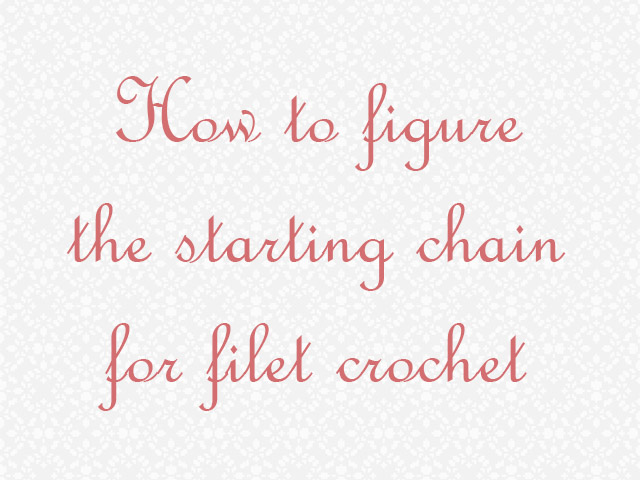# How to figure the starting chain for filet crochetIf you are new to crochet filet world, during the start of your first project you are asking yourself how to figure the starting chain. This count is not difficult and in this simple tutorial i will teach you how to.

Here is how figure how many chains should be in a starting chain for your filet crochet work.

Multiply the number of squares across on the first row of the chart, times 3, then add 1, that is your starting chain, then, if the first square on the chart is a solid mesh, then chain 3, or if the first square on the chart is an open mesh, then chain 5.

Here are two examples with number 3 of squares in the first row:

3×3+1=10 +3=13

3×3+1=10 +5=15